I recently performed some tests in the lab and noticed a strange ringing on the output signal. After troubleshooting the problem, I managed to trace the ringing back to the input signal. Figure 1 shows the input signal of my circuit with the ringing boxed in red. I needed to find the cause of the ringing since a clean step response was expected. It turns out that my lab equipment was not properly terminated. This blog post describes how to properly terminate your lab equipment and the importance of this technique.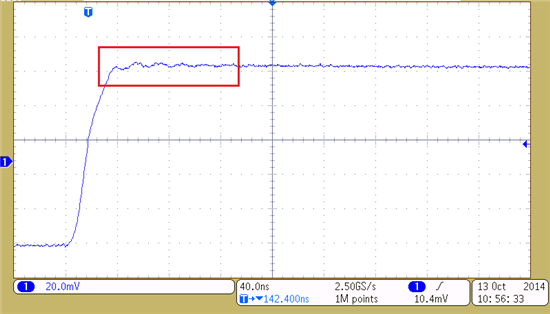Figure 1: Unknown ringing

Figure 2 shows the test circuit with source impedance, ZS, equal to the default 50Ω source impedance of the lab equipment and input impedance Zi.- These can vary depending on what is needed for the circuit.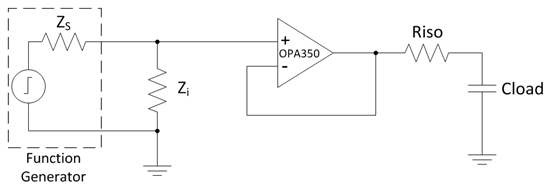Figure 2: Test Circuit

Using the reflection coefficient equation, shown in Equation 1, it is possible to see that if the input impedance is not equal to the source impedance, the reflection coefficient will be a non-zero value.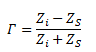Equation 1

Setting Zi which is much greater than ZS, gives a large reflection coefficient. As shown in Equation 2, setting Zi equal to 1MΩ gives a reflection coefficient of approximately 0.9999. This means that almost the entire input signal reflects back to the function generator and results in ringing.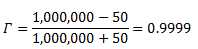Equation 2

Figure 3 shows an oscilloscope shot of a measurement taken at the input of the OPA350 with Zi equal to 1MΩ. This shows a ringing with a peak-to-peak voltage of 4.4mVpp.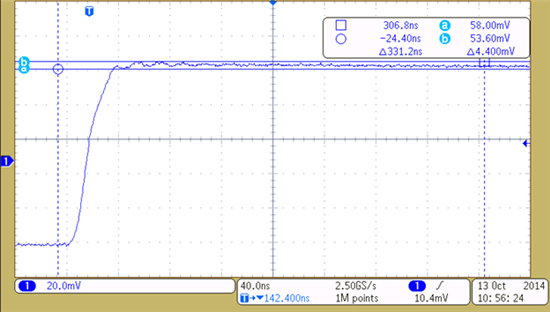Figure 3: Input waveform with Zi=1MΩ

To decrease the peak-to-peak voltage of the oscillations, minimize the reflection coefficient. For example, reducing Zi to 1kΩ, gives a reflection coefficient of 0.905. This will reduce the magnitude of the voltage reflected back to the function generator. Figure 4 shows an oscilloscope shot of a measurement taken at the input of the OPA350 with Zi equal to 1kΩ. Notice the reduction of peak-to-peak voltage to 3.2mVpp.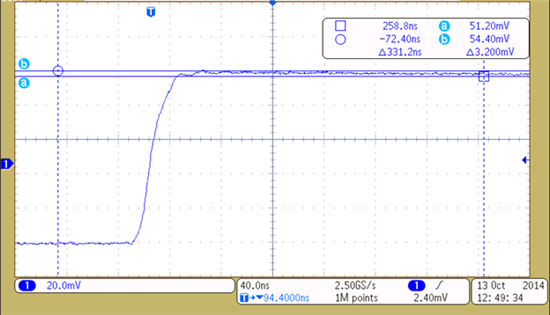Figure 4: Input waveform with Zi=1kΩ

To properly terminate the lab equipment and eliminate the reflections, Zi must be equal to the source impedance of 50Ω. Figure 5 shows a measurement taken at the input of the OPA350 with Zi equal to ZS. Notice how the ringing will stop.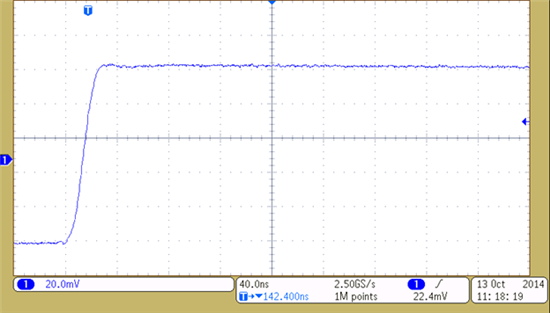Figure 5: Input waveform with Zi=ZS

Properly terminating your lab equipment with a circuit input impedance equal to the source impedance of the equipment is crucial for a clean input signal. Next time you find yourself wondering where an unknown ringing is coming from, remember to check that your lab equipment is properly terminated.

• I think it is important to clarify at which frequencies this effect matters. I know it is important for high frequency circuits (e.g. radio circuits and high speed data buses) and when considering electromagnetic emissions (for CE/FCC compatibility tests), but for low frequency and small signal (e.g. audio aimplifiers), I don't think this impedance adaptation is desired (in fact usually ensuring a high input impedance is usually important).

Otto,

No, placing a capacitor in series with the 50 ohm resistor will not have the

same effect. The capacitor changes the impedance of the termination. The

impedance of a capacitor changes with frequency and therefore as the input

frequency changes so will the termination impedance. You can see the effects

of placing a capacitor in series with a resistor using the free TINA-TI

simulation tool found here. http://www.ti.com/tool/tina-ti

jstoehrer,

Yes you are correct, with a 50 ohm termination the amplitude should be half.

I wanted to emphasize the ringing of the input signal and not how the

amplitude changes. Therefore I used a larger amplitude for the input signal

when using a 50 ohm termination.

Hope this helps!

-Tim Claycomb

• jstoehrer, I suspect that the author changed the load impedance setting on the function generator from High-Z in Figure 3 to 50 Ohms in Figure 5.  The function generator automatically adjusts its output so that you view a full-scale waveform on the scope.

• With 50 ohm termination, the amplitude should drop to half the value of  Zi equal to 1MΩ.

Why is it always the same in your screen shots?

• High Timothy!

Thank you for your nice article. It is always interesting when at an unexpected occasion it turns out that the electromagnetism propagates. I would like to add a small clarification. Not (only) the lab equipment must be terminated but the cable between the lab equipment and the DUT. If you vary the length of the poorly terminated cable you will see that the frequency of the ringing changes.

Thank you again,

Gábor